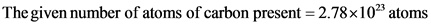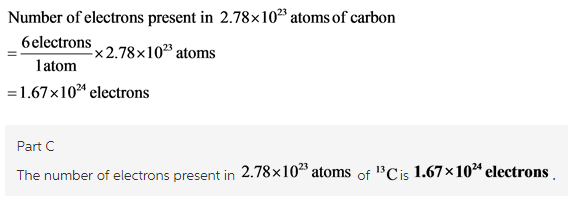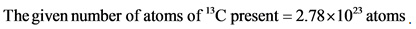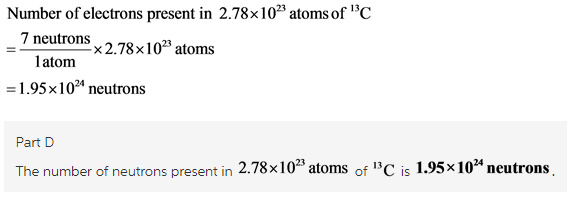# Based on your answer in Part B, how many electrons are in this amount of 13C?

Part C
Based on your answer in Part B, how many electrons are in this amount of13C ?

Part B Answer: 2.78*10^23 atoms of carbon

Part D
Based on your answer in Part B, how many neutrons are in this amount of 13C?

Part B Answer: 2.78*10^23 atoms of carbon

Concepts and reason
The number of atoms of carbon present in a sample is given. To calculate the number of electrons and neutrons present in that number of carbon atoms, first find the number of electrons and neutrons present in one carbon atom, that is,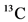by using its atomic number and mass number. Then, obtain the conversion factor for each and calculate the same.

Fundamentals
The number of electrons present in an atom is equal to the number of protons to maintain the electrical neutrality of an atom. The atomic number of an atom represents the number of protons present. Thus,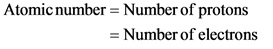Similarly, the number of neutrons present in an atom can be calculated by the mass number. Consider the following relation: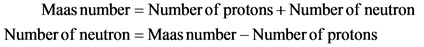Isotopes have different mass number and same atomic number. Therefore, in isotopes mass number varies due to presence of extra neutrons.